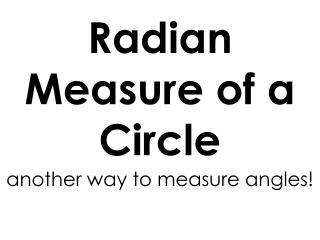Download Presentation# Radian Measure of a Circle

Download Presentation## Radian Measure of a Circle

- - - - - - - - - - - - - - - - - - - - - - - - - - - E N D - - - - - - - - - - - - - - - - - - - - - - - - - - -
##### Presentation Transcript

1. Radian Measure of a Circle another way to measure angles!

2. Opener: • What is the circumference of a tire with a radius of 18”? • How many complete revolutions does the tire make over 1000 feet?

3. Measuring Angles and Arcs • An object has been chosen for you. • You will be working as a group. • Choose a member to do a specific job: measurer(2), recorder, calculator.

4. Measuring Circular Objects • While someone holds the object, measure the diameter to the nearest 1/8 of an inch. The most accurate measurement can be obtained by securing one end and moving the other to get the largest measurement as the diameter is the chord of greatest length.

5. Measuring Circular Objects • Once the diameter has been obtained, determine the radius of the object (without measuring). • Afterwards, we will use our tape measure to determine the circumference of the object. What is circumference a measure of???

6. Measuring Circular Objects • Hold the end securely and wrap the tape measure around the object to measure circumference. Once again measure to the nearest 1/8 of an inch. • Record this measurement.

7. Measuring Circular Objects • Use the radius you calculated earlier to determine how many radii will fit around the outside of the object. • Record your answer. This number correlates to the “radians” in a circle.

8. Measuring Circular Objects • Divide the number of radii that fit around your object by π. Record you answer to three decimal places. • Compare your answer with those from the groups around you. Are they the same? Should they be?

9. Measuring Circular Objects • How many radii would it take to fit half way around your object? • You and your group need to come up with an explanation of what π represents and record your answer.

10. Measuring Circular Objects • How many radii does it take to fit around the entire object? Divide by π to determine how many radii in terms of π. How many degrees is it all the way around the object? • Come up with a conversion factor to convert from degrees to radians using π.

11. How to convert from … • Degrees to Radians: • Radians to Degrees: